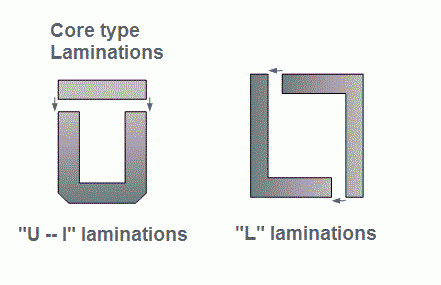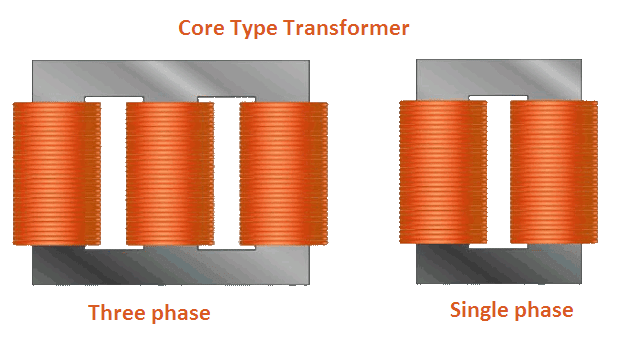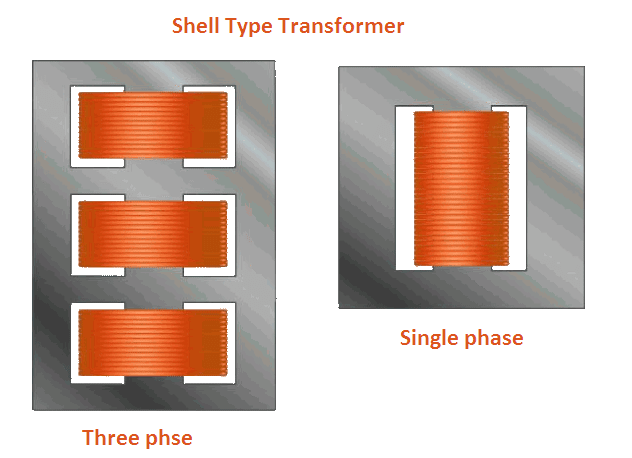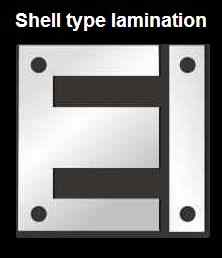# Types of Transformer

There are many types of transformer. These can be classified on the different basis. Following are the main types of transformer.

# Types of Transformer on the Basis of Core Arrangement

On the basis of core and winding arrangement, following are the main types of transformer:

• Core type transformer
• Shell type transformer
• Berry type transformer

## Core Type Transformer

In the core type transformer magnetic core is made from ‘L’ shape strips. The core type transformer consists of an iron core surrounded by windings. The core of this transformer has two limbs.

Each limb carries the same flux. Therefore, the area of both limbs is equal.

The low voltage winding is placed next to the core and high voltage winding is placed over it. Proper insulation is provided in between core, low voltage winding and high voltage windings.## Shell Type TransformerIn the core type transformer, the iron core is surrounded by the windings whereas, in the shell type transformer, the windings are surrounded by the iron core. The core of shell type transformer is made from ‘E’ and ‘I’ strips.The laminations of this transformer have three limbs. The side limbs carry half of the flux whereas central limb carries the whole of the flux. Therefore, the width of the central limb is more than that of the outer limbs.

Both the windings are placed on the central limb concentrically or side by side. The low voltage winding is placed next to the core and high voltage winding is placed over it. Proper insulation is provided in between core, low voltage winding and high voltage windings.

## Types of Transformer on the Basis of Transformation Ratio

The transformation ratio of the transformer may be defined as the ratio of the secondary voltage to the primary voltage of the transformer. It is denoted by K.

Therefore, transformation ratio K = V2/V1

And K = E2/E1 = N2/N1 = I1/I2 also.

Where V1, E1, N1 are the supply voltage, induced EMF, winding turns of the primary winding respectively. And V2, E2, N2 are output voltage, induced EMF, winding turns of secondary winding respectively.

On the basis of the value of K, types of transformers are as follows:

## Step-up Transformer

The transformers having K > 1 i.e. N2 > N1 are known as the step-up transformer. In such type of transformers, we get high output voltage as compared to the applied voltage, hence the name step-up.

## Step-down Transformer

The transformers having K < 1 i.e. N2 < N1 are known as the step-down transformer. In such type of transformers, we get low output voltage as compared to the applied voltage, hence the name step-down.

## One-to-one Transformer

The transformers having K = 1 i.e. N2 = N1 are known as the one-to-one transformer. In such type of transformers output voltage is equal to the input voltage. The main function of such transformer is to provide isolation between input and output circuit, hence this transformer is also known as the isolation transformer.

## Types of Transformer on the Basis of Applications

The transformers are often classified on the basis of the purposes for which they are used. Following are the important types of transformer on the basis of applications:

## Power Transformers

These transformers are used at the power plants to step-up the generated voltage for transmission purposes and to step down the voltage at the receiving substations. These are large size transformers.

These transformers are usually operated near the full load which would cause high copper loss. Thus, to have minimum losses, such transformers are designed with low copper losses and to give maximum efficiency at near the full load.

## Distribution Transformers

These transformers are used to step down the voltage at the distribution substations. The load on such transformers varies for all the 24 hours from no load to full load.

Such transformers are designed with low iron loss and to give maximum efficiency at about 75% of the full load to obtain high efficiency
.

Autotransformers

## Instrument Transformers

These transformers are used to measure high voltage, high current and to operate protective devices. To measure high voltage, it is stepped down with an instrument transformer which is known as the potential transformer (PT) and then applied to a voltmeter. Similarly, to measure high current, it is stepped down with an instrument transformer which is known as the current transformer (CT) and then applied to an ammeter.

## Testing Transformers

To carry out the tests under high, voltages testing transformers are used to step-up the voltage to a very high value.

## Special Transformers

These are used to operate welding sets, rectifiers, electric furnaces etc.

## Transformer | All Posts

//www.yourelectricalguide.com/ types of transformer.